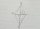# Trapezoid + expression of a variable from formula - problems

Trapezoid is a convex quadrilateral with one pair of parallel sides, but referred as a trapezium outside North America. The parallel sides are called the bases of the trapezoid and the other two sides are called the legs or the lateral sides.

1. A kiteABCD is a kite. Angle OBC = 20° and angle OCD = 35°. O is the intersection of diagonals. Find angle ABC, angle ADC and angle BAD.
2. Rectangular trapezoidIn a rectangular trapezoid ABCD with right angles at vertices A and D with sides a = 12cm, b = 13cm, c = 7cm. Find the angles beta and gamma and height v.
3. IS trapezoidCalculate the length of diagonal u and height v of isosceles trapezoid ABCD, whose bases have lengths a = |AB| = 37 cm, c = |CD| = 29 cm and legs b = d = |BC| = |AD| = 28 cm.
4. R TrapeziumRectangular trapezium has bases 12 and 5 and area 84 cm2. What is its perimeter?
5. GardenTrapezoid garden has parallel sides 19 m and 24 m. Its area is 193.5 square meters. What is the width of the garden?
6. Trapezoidtrapezoid ABCD a = 35 m, b=28 m c = 11 m and d = 14 m. How to calculate its area?
7. Rectangular trapezoidThe rectangular trapezoid ABCD is: /AB/ = /BC/ = /AC/. The length of the median is 6 cm. Calculate the circumference and area of a trapezoid.
8. Trapezoid ABCD v2Trapezoid ABCD has length of bases in ratio 3:10. The area of riangle ACD is 825 dm2. What is the area of trapezoid ABCD?The quadrangular prism has a volume 648 cm3. Trapezoid which is its base has the dimensions bases: a = 10 cm, c = 5 and height v = 6 cm. What is the height of the prism?
10. TrapezoidArea of trapezoid is 135 cm2. Sides a, c and height h are in a ratio 6:4:3. How long are a,c and h? Make calculation...
11. Trapezoid thirdsThe ABCD trapezoid with the parallel sides of the AB and the CD and the E point of the AB side if the segment DE divides the trapezoid into two parts with the same area. Find the length of the AE line segment.
12. Rectangular trapeziumCalculate the perimeter of a rectangular trapezium when its content area is 576 cm2 and sice a (base) is 30 cm, height 24 cm.
13. Trapezoid 15Area of trapezoid is 266. What value is x if bases b1 is 2x-3, b2 is 2x+1 and height h is x+4
14. Isosceles trapezoidWhat is the height of an isosceles trapezoid, the base of which has a length of 11 cm and 8 cm and whose legs measure 2.5 cm?
15. Isosceles trapezoidCalculate the content of an isosceles trapezoid whose bases are at ratio 5:3, the arm is 6cm long and it is 4cm high.
16. TrapezoidHow long are the trapezoid bases with area 24 cm2 and height 3 cm. One base is 3 times longer than the shorter.
17. Water channelThe cross section of the water channel is a trapezoid. The width of the bottom is 19.7 m, the water surface width is 28.5 m, the side walls have a slope of 67°30' and 61°15'. Calculate how much water flows through the channel in 5 minutes if the water flow
18. Trapezoid MO-5-Z8Trapezoid KLMN has bases 12 and 4 cm long. The area of triangle KMN is 9 cm2. What is the area of the trapezoid KLMN?
19. Land - isosceles trapezoidCalculate the content and perimeter of the building plot in the form of an isosceles trapezoid with bases 120m, 95m and height 50m.
20. TrenchThe trench is a four-sided prism. The cross section has a trapezoidal shape with basements of 4m and 6m, the length of the trench is 30m. What is the depth of the trench if we dig 60,000 l of soil.

Do you have an interesting mathematical problem that you can't solve it? Enter it, and we can try to solve it.

To this e-mail address, we will reply solution; solved examples are also published here. Please enter e-mail correctly and check whether you don't have a full mailbox.